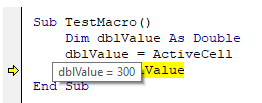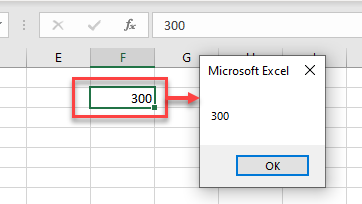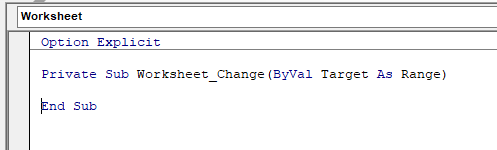# VBA Set ActiveCell

This tutorial will demonstrate how to set (and work with) the ActiveCell using VBA.

The ActiveCell property in VBA returns the address of the cell that is selected (active) in your worksheet. We can move the cell pointer to a specific cell using VBA by setting the ActiveCell property to a specific cell, and we can also read the values of the currently active cell with VBA.

## Set ActiveCell

Setting the active cell in VBA is very simple – you just refer to a range as the active cell.

``````Sub Macro1()
ActiveCell = Range("F2")
End Sub``````

This will move your cell pointer to cell F2.

## Get Value of ActiveCell

We can get the value of an active cell by populating a variable.

For example, if the value in F2 if 300, we can return this value to a declared variable.

``````Sub TestMacro()
Dim dblValue As Double
dblValue = ActiveCell
MsgBox dblValue
End Sub``````

when we run the code, the variable dblValue will be populated with the value in the ActiveCell.If we then allow the code to continue, a message box will pop up with the value.## Get ActiveCell in Worksheet_Change Event

When you change any data in your worksheet, the Worksheet_Change Event is fired.The Change event contains one argument  –  (ByVal Target as Range). The Range referred to in this variable Target is either a range of cells or a single cell that is currently selected in your worksheet. If you have only one cell selected in the worksheet, then the Target variable is equal to the ActiveCell.

``````Private Sub Worksheet_Change(ByVal Target As Range)
If Target = Range("F2") Then
MsgBox "The Active cell is F2!"
End If
End Sub``````

Note that this event only fires when you amend the data in your worksheet – so when you add or change data, it does not fire by moving your cell pointer around the worksheet.